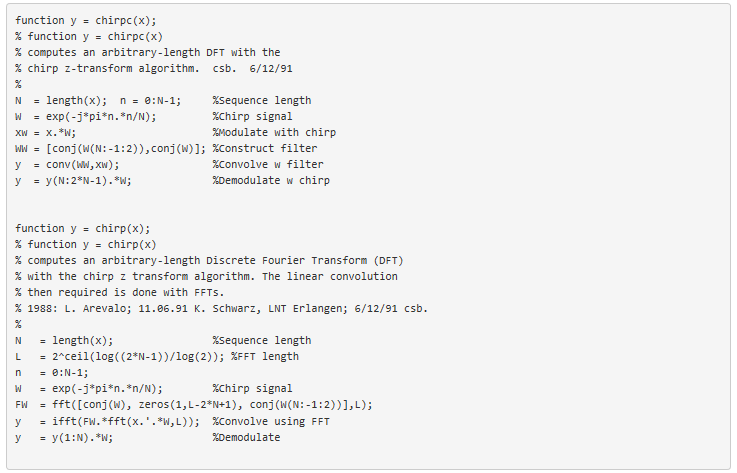# 4.3: The Chirp Z-Transform or Bluestein's Algorithm

The DFT of $$x(n)$$ evaluates the $$\mathit{Z}$$-transform of $$x(n)$$ on $$N$$ equally spaced points on the unit circle in the $$\mathit{z}$$ plane. Using a nonlinear change of variables, one can create a structure which is equivalent to modulation and filtering $$x(n)$$ by a “chirp" signal.

The mathematical identity

$(k-n)^{2}=k^{2}-2kn+n^{2}$

gives

$nk=\frac{\left ( n^{2}-(k-n)^{2}+k^{2}\right )}{2}$

which substituted into the definition of the DFT in Multidimensional Index Mapping Equation gives

$C(k)=\left \{ \sum_{n=0}^{N-1} [x(n)W^{n^{2}/2}]W^{-(k-n)^{2}/2}\right \}W^{k^{2}/2}$

This equation can be interpreted as first multiplying (modulating) the data $$x(n)$$ by a chirp sequence $$W^{n^{2}/2}$$ then convolving (filtering) it, then finally multiplying the filter output by the chirp sequence to give the DFT.

Define the chirp sequence or signal as

$h(n)=W^{n^{2}/2}$

which is called a chirp because the squared exponent gives a sinusoid with changing frequency. Using this definition, we have:

$C(n)=\left \{ [x(n)h(n)]\ast h^{-1}\right \}h(n)$

We know that convolution can be carried out by multiplying the DFTs of the signals, here we see that evaluation of the DFT can be carried out by convolution. Indeed, the convolution represented by $$\ast \ast$$ in the equation can be carried out by DFTs (actually FFTs) of a larger length. This allows a prime length DFT to be calculated by a very efficient length- $$2^M$$ FFT. This becomes practical for large $$N$$ when a particular non-composite (or $$N$$ with few factors) length is required.

As developed here, the chirp $$\mathit{z}$$-transform evaluates the $$\mathit{z}$$-transform at equally spaced points on the unit circle. A slight modification allows evaluation on a spiral and in segments and allows savings with only some input values are nonzero or when only some output values are needed.

Two Matlab programs to calculate an arbitrary length DFT using the chirp $$\mathit{z}$$-transform is shown in Pre.## Contributor

• ContribEEBurrus